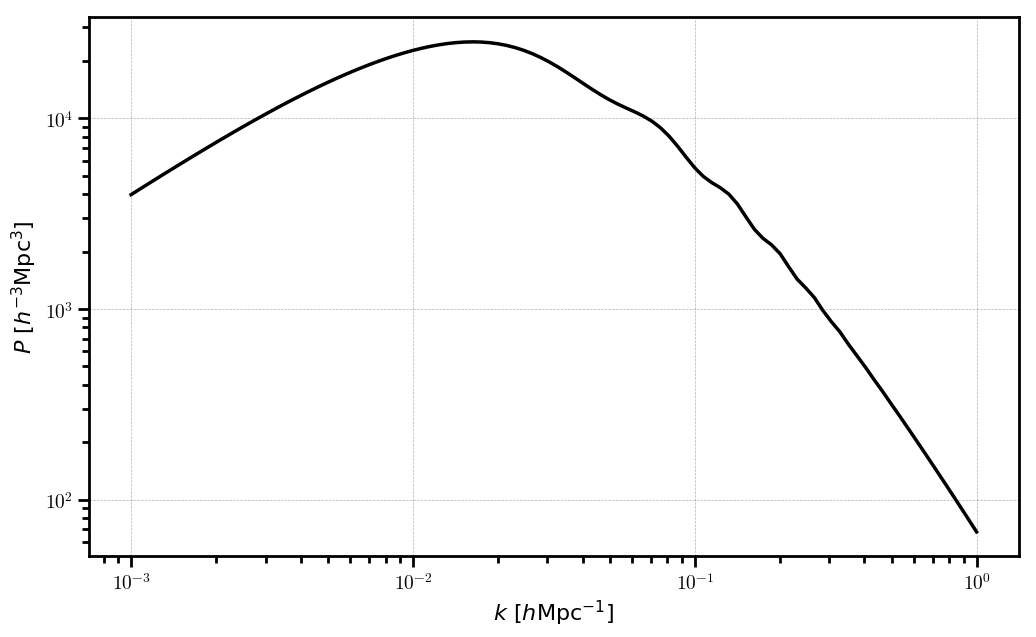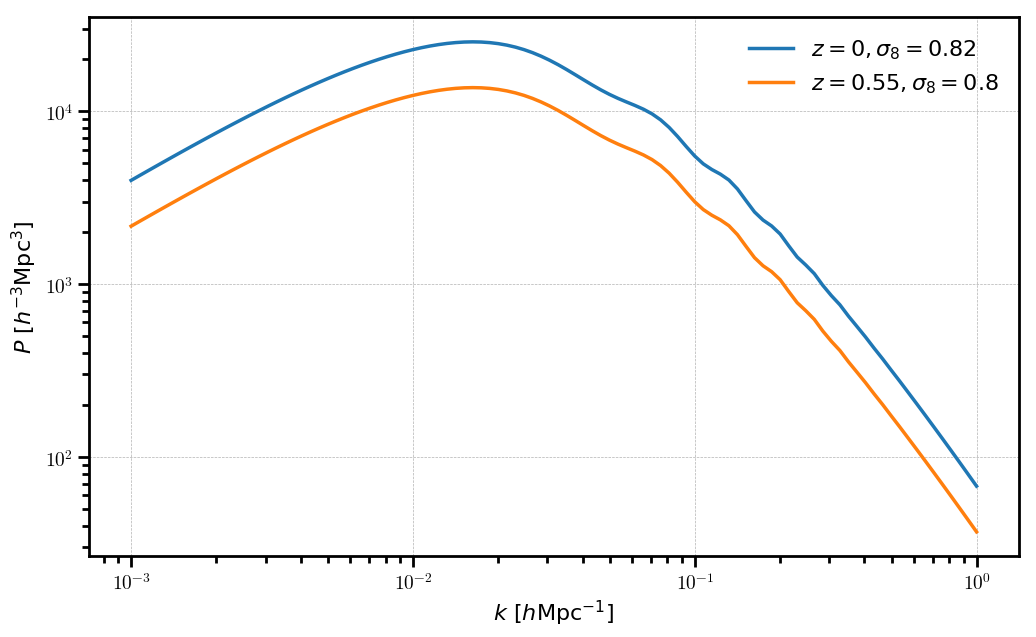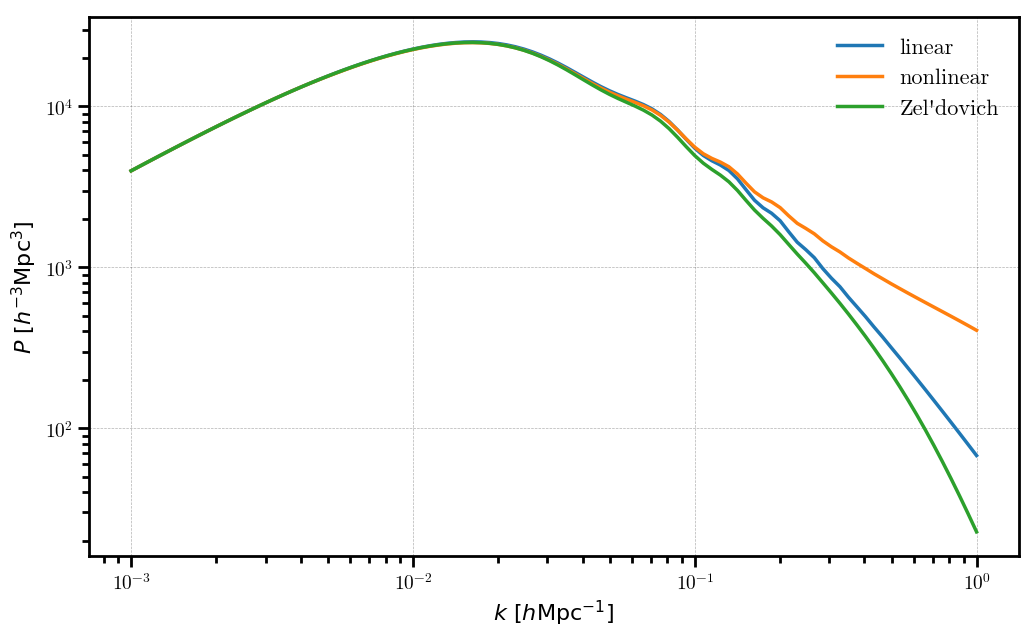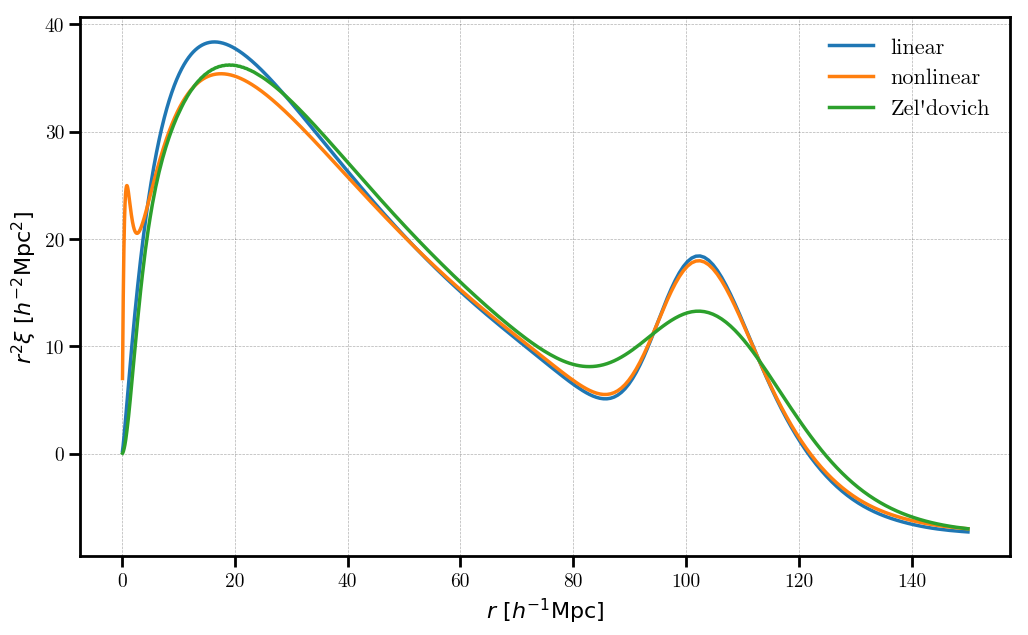# Cosmological Calculations¶

The nbodykit.cosmology module includes functionality for representing cosmological parameter sets and computing various theoretical quantities prevalent in large-scale structure that depend on the background cosmological model. The module relies on the CLASS CMB Boltzmann code as the underlying engine for its cosmological calculations via the Python wrapper provided by the classylss package. For consistency, the cosmology syntax used in nbodykit largely follows that of the CLASS code. A useful set of links for reference material on CLASS is available here.

## The Cosmology class¶

nbodykit provides the Cosmology class for representing cosmological parameter sets. Given an input set of parameters, this class uses the CLASS code to evaluate various quantities relevant to large-scale structure. The class can compute background quantities, such as distances, as a function of redshift, as well as linear and nonlinear power spectra and transfer functions.

Note

For a full list of the attributes and functions available, please see the API docs.

The CLASS code supports multiple sets of input parameters, e.g., the user can specify H0 or h. For simplicity, we use the following parameters to initialize a Cosmology object:

• h : the dimensionless Hubble parameter
• T0_cmb : the temperature of the CMB in Kelvins
• Omega0_b : the current baryon density parameter, $$\Omega_{b,0}$$
• Omega0_cdm : the current cold dark matter density parameter, $$\Omega_{cdm,0}$$
• N_ur : the number of ultra-relativistic (massless neutrino) species
• m_ncdm : the masses (in eV) for all massive neutrino species
• P_k_max : the maximum wavenumber to compute power spectrum results to, in units of $$h \mathrm{Mpc}^{-1}$$
• P_z_max : the maximum redshift to compute power spectrum results to
• gauge : the General Relativity gauge, either “synchronous” or “newtonian”
• n_s : the tilt of the primordial power spectrum
• nonlinear : whether to compute nonlinear power spectrum results via HaloFit

Any valid CLASS parameters can be additionally specified as keywords to the Cosmology constructor. If these additional parameters conflict with the base set of parameters above, an exception will be raised. For a guide to the valid input CLASS parameters, the “explanatory.ini” parameter file in the CLASS GitHub is a good place to start.

Notes and Caveats

• The default configuration assumes a flat cosmology, $$\Omega_{k,0}=0$$. Pass Omega0_k as a keyword to specify the desired non-flat curvature.
• By default, the value for N_ur is inferred from the number of massive neutrinos using the following logic: if you have respectively 1,2,3 massive neutrinos and use the default T_ncdm value (0.71611 K), which is designed to give m/omega of 93.14 eV, and you wish to have N_eff=3.046 in the early universe, then N_ur is set to 2.0328, 1.0196, 0.00641, respectively.
• For consistency of variable names, the present day values can be passed with or without the “0” postfix, e.g., Omega0_cdm is translated to Omega_cdm as CLASS always uses the names without “0” as input parameters.
• By default, a cosmological constant (Omega0_lambda) is assumed, with its density value inferred by the curvature condition.
• Non-cosmological constant dark energy can be used by specifying the w0_fld, wa_fld, and/or Omega0_fld values.
• To pass in CLASS parameters that are not valid Python argument names, use the dictionary/keyword arguments trick, e.g., Cosmology(..., **{'temperature contributions': 'y'})

### Builtin Cosmologies¶

We include several builtin cosmologies for users, accessible from the nbodykit.cosmology module:

Name Source $$H_0$$ $$\Omega_{m,0}$$ Flat
WMAP5 Komatsu et al. 2009 70.2 0.277 Yes
WMAP7 Komatsu et al. 2011 70.4 0.272 Yes
WMAP9 Hinshaw et al. 2013 69.3 0.287 Yes
Planck13 Planck Collab 2013, Paper XVI 67.8 0.307 Yes
Planck15 Planck Collab 2015, Paper XIII 67.7 0.307 Yes

### Updating cosmological parameters¶

The Cosmology class is immutable. To update cosmological parameters, we provide the following functions:

• clone(): provides a new Cosmology object with only the specified parameters changed; this function accepts any keyword parameters that can be passed to the Cosmology constructor
• match(): provides a new Cosmology object that has matched a specific, derived parameter value. The derived parameters that can be matched include:
• sigma8: re-scale the scalar amplitude A_s to match sigma8
• Omega0_cb: match the total sum of cdm and baryons density, $$\Omega_{cdm,0}+\Omega_{b,0}$$
• Omega0_m: match the total density of matter-like components, $$\Omega_{cdm,0}+\Omega_{b,0}+\Omega_{ncdm,0}-\Omega_{pncdm,0}+\Omega_{dcdm,0}$$

For example, we can match a specific sigma8 value using:

In :

from nbodykit.lab import cosmology

cosmo = cosmology.Cosmology()
print("original sigma8 = %.4f" % cosmo.sigma8)

new_cosmo = cosmo.match(sigma8=0.82)
print("new sigma8 = %.4f" % new_cosmo.sigma8)

original sigma8 = 0.8380
new sigma8 = 0.8200


And we can clone a Cosmology using

In :

cosmo = cosmo.clone(h=0.7, nonlinear=True)


### Converting to a dictionary¶

The keyword parameters passed to the constructor of a Cosmology object are not passed directly to the underlying CLASS engine, but must be edited first to be compatible with CLASS. Users can access the full set of parameters passed directly to CLASS by converting the Cosmology object to a dict. This can be accomplished simply using:

In :

cosmo_dict = dict(cosmo)
print(cosmo_dict)

{'output': 'vTk dTk mPk', 'extra metric transfer functions': 'y', 'n_s': 0.9667, 'gauge': 'synchronous', 'N_ur': 2.0328, 'T_cmb': 2.7255, 'Omega_cdm': 0.26377065934278865, 'Omega_b': 0.0482754208891869, 'N_ncdm': 1, 'P_k_max_h/Mpc': 10.0, 'z_max_pk': 100.0, 'h': 0.7, 'm_ncdm': [0.06], 'non linear': 'halofit'}


Each of the parameters in this dictionary are valid CLASS parameters. For example, we see that the CLASS parameter “non linear” has been set to “halofit”, since we have set the nonlinear parameter to be True.

Note

To create a new Cosmology object from a dictionary, users should use Cosmology.from_dict(dict(c)). Because of the differences in syntax between the Cosmology constructor and CLASS, the following will not work: Cosmology(**dict(c)).

### Interfacing with astropy¶

We provide some comptability for interfacing with the astropy.cosmology module. The following functions of the Cosmology() class allow users to convert between the nbodykit and astropy cosmology implementations:

 to_astropy() Initialize and return a subclass of astropy.cosmology.FLRW from the Cosmology class. from_astropy(cosmo, **kwargs) Initialize and return a Cosmology object from a subclass of astropy.cosmology.FLRW.

We also provide support for some of the syntax used by astropy for attributes in its cosmology classes by aliasing the astropy names to the appropriate nbodykit attributes. For example,

 Om0 Returns Omega0_m. Odm0 Returns Omega0_cdm. Ode0 Returns the sum of Omega0_lambda and Omega0_fld. Ob0 Returns Omega0_b.

Warning

The conversion between astropy and nbodykit cosmology objects is not one-to-one, as the classes are implemented using different underlying engines. For example, the neutrino calculations performed in nbodykit using CLASS differ from those in astropy. We have attempted to provide as much compatibility as possible, but these differences will always remain when converting between the two implementations.

## Theoretical Power Spectra¶

### Linear power¶

The linear power spectrum can be computed for a given Cosmology and redshift using the LinearPower class. This object can compute the linear power spectrum using one of several transfer functions:

• “CLASS”: calls CLASS to evaluate the total density transfer function at the specified redshift
• “EisensteinHu”: uses the analytic transfer function from Eisenstein & Hu, 1998
• “NoWiggleEisensteinHu”: uses the no-BAO analytic transfer function from Eisenstein & Hu, 1998

The LinearPower object is a callable function, taking wavenumber in units of $$h \mathrm{Mpc}^{-1}$$ as input:

In :

import matplotlib.pyplot as plt
import numpy as np

c = cosmology.Planck15
Plin = cosmology.LinearPower(c, redshift=0., transfer='CLASS')

k = np.logspace(-3, 0, 100)
plt.loglog(k, Plin(k), c='k')

plt.xlabel(r"$k$ $[h \mathrm{Mpc}^{-1}]$")
plt.ylabel(r"$P$ $[h^{-3} \mathrm{Mpc}^{3}]$")
plt.show()The redshift and power spectrum normalization can easily be updated by adjusting the redshift and sigma8 attributes of the LinearPower instance. For example,

In :

# original power
plt.loglog(k, Plin(k), label=r"$z=0, \sigma_8=%.2f$" % Plin.sigma8)

# update the redshift and sigma8
Plin.redshift = 0.55
Plin.sigma8 = 0.80
plt.loglog(k, Plin(k), label=r"$z=0.55, \sigma_8=0.8$")

# format the axes
plt.legend()
plt.xlabel(r"$k$ $[h \mathrm{Mpc}^{-1}]$")
plt.ylabel(r"$P$ $[h^{-3} \mathrm{Mpc}^{3}]$")
plt.show()### Nonlinear and Zel’dovich power¶

We also provide the HalofitPower and ZeldovichPower classes to compute the nonlinear and Zel’dovich power spectra, respectively. The nonlinear power uses the HaloFit prescription that is available in CLASS. These objects behavior very similarly to the LinearPower class. For example,

In :

# initialize the power objects
Plin = cosmology.LinearPower(c, redshift=0, transfer='CLASS')
Pnl = cosmology.HalofitPower(c, redshift=0)
Pzel = cosmology.ZeldovichPower(c, redshift=0)

# plot each kind
plt.loglog(k, Plin(k), label='linear')
plt.loglog(k, Pnl(k), label='nonlinear')
plt.loglog(k, Pzel(k), label="Zel'dovich")

# format the axes
plt.legend()
plt.xlabel(r"$k$ $[h \mathrm{Mpc}^{-1}]$")
plt.ylabel(r"$P$ $[h^{-3} \mathrm{Mpc}^{3}]$")
plt.show()## Correlation functions¶

nbodykit also includes functionality for computing theoretical correlation functions by computing the Fourier transform of a power spectrum. We use the mcfit package, which provides a Python version of the FFTLog algorithm. The main class to compute correlation functions is CorrelationFunction. This class takes a power spectrum instance (one of LinearPower, HalofitPower, or ZeldovichPower) as input. Below, we show the correlation function for each of these classes:

In :

# initialize the correlation objects
cf_lin = cosmology.CorrelationFunction(Plin)
cf_nl = cosmology.CorrelationFunction(Pnl)
cf_zel = cosmology.CorrelationFunction(Pzel)

# plot each kind
r = np.logspace(-1, np.log10(150), 1000)
plt.plot(r, r**2 * cf_lin(r), label='linear')
plt.plot(r, r**2 * cf_nl(r), label='nonlinear')
plt.plot(r, r**2 * cf_zel(r), label="Zel'dovich")

# format the axes
plt.legend()
plt.xlabel(r"$r$ $[h^{-1} \mathrm{Mpc}]$")
plt.ylabel(r"$r^2 \xi \ [h^{-2} \mathrm{Mpc}^2]$")
plt.show()We also include utility functions for performing the $P(k) leftrightarrow xi(r)$ transformation:

 xi_to_pk(r, xi[, extrap]) Return a callable function returning the power spectrum, as computed from the Fourier transform of the input $$r$$ and $$\xi(r)$$ arrays. pk_to_xi(k, Pk[, extrap]) Return a callable function returning the correlation function, as computed from the Fourier transform of the input $$k$$ and $$P(k)$$ arrays.

These functions take discretely evaluated $$(r, \xi(r))$$ or $$(k, P(k))$$ arrays and return a function that evaluates the appropriate transformed quantity.